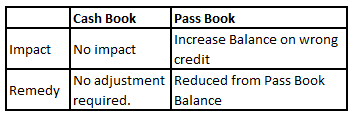# Bank Reconciliation Statement Questions | Accounts Class 11

Bank Reconciliation Statement Questions can be divided into the following four categories  : –

• There is a Debit  balance in the books of accounts, and a debit  balance as per the bank passbook
• There is a Debit  balance in the books of accounts, and a  credit balance as per the bank passbook
• There is a  credit balance in the books of accounts, and a debit   balance as per the bank passbook
• There is a  credit balance in the books of accounts, and a  credit balance as per the bank passbook
In this post we are going to look at Bank Reconciliation Statement Questions, solutions and reasoning for solutions,  when we have a Favorable or Credit Balance as per the Passbook .

Bank Reconciliation Statement Question 1:

#### Favourable balance as per the pass book – Cheque deposited but not collected (credited) by the bank

If the Balance as per Pass Book is a (Cr.) of Rs. 15300 , cheque of Rs. 5000 deposited into the Bank,but not collected by Bank would result an/a ___________ in Cr. balance ?

Explanation:

When a Cheque is deposited into the bank, but not credited by the bank, the balance as per the bank Pass Book would remain the same.

However, if such cheque deposit is recorded in the cash book, the cash book balance would increase.

Cheque deposited into the bank would be added to the Pass Book Balance to bring it at equal to the balance as per the Cash book.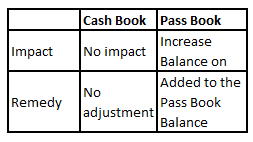#### Favourable balance as per the pass book- Deposited cheque or bill of exchange dishonoured

If the Balance as per Pass Book is a (Cr.) of Rs. 16000 , cheque of Rs. 7500 which is dishonoured due to insufficient fund would result an/a __________ in Cr. balance ?

Explanation:

When a Cheque or bill of exchange is dishonoured, the balance as per the Pass Book would have remained the same and not increased.

However, as such cheque is considered as collected, it would have increased the Cash book balance.

To reconcile the two, such deposit would be added to the Pass Book balance to bring it at par with balance as per the Cash book.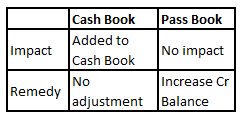Bank Reconciliation Statement Question 3:

#### Favourable balance as per the pass book – bank charges

If the Balance as per Pass Book is a (Cr.) of Rs. 11500 , Bank charges of Rs. 2000 not recorded in the Cash Book would result an/a ___________ in Cr. balance ?

Explanation:

When the Bank Debits Bank charges, the balance as per the Pass Book would have reduced.

However, as such charges are not considered in Cash Book, the Cash book balance would have remained the same.

To reconcile the two, such charges would be added to the Pass Book balance to bring it at par with balance as per the Cash book.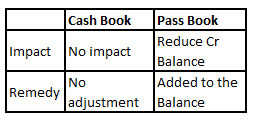#### Favourable balance as per the pass book- interest charged by the bank

If the Balance as per Pass Book is a (Cr.) of Rs. 11900 , interest charges of Rs. 350 of Bank not recorded in the Cash Book would result an/a ______________ in Cr. balance ?

Explanation:

When the Bank Debits Interest, the balance as per the Pass Book would have reduced.

However, as such interest charges are not considered in Cash Book, the Cash book balance would have remained the same.

To reconcile the two, such interest charges would be added to the Pass Book balance to bring it at par with balance as per the Cash book.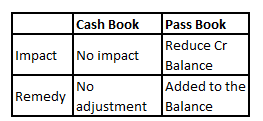Bank Reconciliation Statement Question 5 :

#### Favourable balance as per the pass book-bill payable paid by the bank but not recorded in the cash book

If the Balance as per Pass Book is a (Cr.) of Rs. 11000 , Bill Payable of Rs. 27500 paid by the Bank but not recorded in the Cash Book would result an/a ___________ in Cr. balance ?

Explanation:

When the Bank Pays Bill Payable, the balance as per the Pass Book would have reduced.

However, as such payment are not considered in Cash Book, the Cash book balance would have remained the same.

To reconcile the two, the payment would be added to the Pass Book balance to bring it at par with balance as per the Cash book.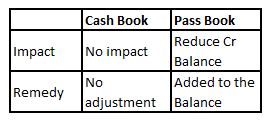Bank Reconciliation Statement Question 6 :

#### Favourable balance as per the pass book-cheque recorded but not deposited

If the Balance as per Pass Book is a (Cr.) of Rs. 12500 , cheque of Rs. 2000 recorded in the Cash Book but not deposited in Bank would result an/a ____________ in Cr. balance ?

Explanation:

When the Cheques are recorded in the books of account, but not deposited , the debit balance as per the Cash Book would have increased.

However, as such debits are not considered in Pass Book, the Pass book balance would have remained the same.

To reconcile the two, such cheques would be added to the Pass Book balance to bring it at par with balance as per the Cash book.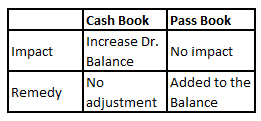Bank Reconciliation Statement Question 7 :

#### Favourable balance as per the pass book-direct payment made by the bank

If the Balance as per Pass Book is a (Cr.) of Rs. 11000 , direct payment of Rs. 2000 by the Bank would result an/a ___________ in Cr. balance ?

Explanation:

When the Bank makes direct payments, the balance as per the Pass Book would have reduced.

However, as such payments are not considered in Cash Book, the Cash book balance would have remained the same.

To reconcile the two, such payment would be added to the Pass Book balance to bring it at par with balance as per the Cash book.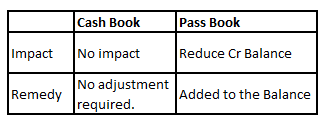#### Favourable balance as per the pass book-wrongly debited by the bank

If the Balance as per Pass Book is a (Cr.) of Rs. 15000 , amount of Rs. 3000 wrongly debited by the Bank would result an/a _____________ in Cr. balance ?

Explanation:

When the Bank makes wrong debit, the balance as per the Pass Book would have reduced.

However, as such debits are not considered in Cash Book, the Cash book balance would have remained the same.

To reconcile the two, such charges would be added to the Pass Book balance to bring it at par with balance as per the Cash book.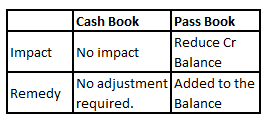#### Favourable balance as per the pass book-cheque issued but not presented for payment

If the Balance as per Pass Book is a (Cr.) of Rs. 10000 , cheque of Rs. 6000 issued, but not presented for the payment would result an/a ___________ in Cr. balance ?

Explanation:

When a Cheques is issued by the company, it reduces it from its Cash Book Balance. However, if such cheque is not presented for payment , this implies that the same has not been reduced by the Bank from its Pass Book balance.

Cheques issued but not presented for payment , would be reduced from the Pass Book balance. This would make the balance as per Pass Book to an amount, which would have been if such cheque were presented for Payment.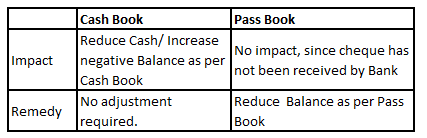Bank Reconciliation Statement Question 10 :

#### Favourable balance as per the pass book-cheque directly deposited into bank

If the Balance as per Pass Book is a (Cr.) of Rs. 11200 , cheque of Rs. 9700 directly deposited by a customer into the Bank would result an/a _____________ in Cr. balance ?

Explanation:

When a Cheque is directly deposited by a debtor into the bank, the balance as per the bank would increase on collection of such cheque.

However, if such cheque deposit is not recorded in the cash book, the cash book balance would not be impacted.
Since cheque deposit would increase Bank balance, Cheque directly deposited by a debtor into the bank would be reduced from Pass Book Balance to bring it at equal to the balance as per the cash book.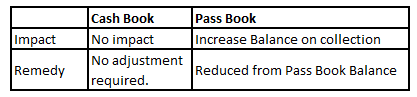#### Favourable balance as per the pass book-cheque recorded but not deposited

If the Balance as per Pass Book is a (Cr.) of Rs. 12500 , cheque of Rs. 2000 recorded in the Cash Book but not deposited in Bank would result an/a _____________ in Cr. balance ?

Explanation:

When the Cheques are recorded in the books of account, but not deposited , the debit balance as per the Cash Book would have increased.

However, as such debits are not considered in Pass Book, the Pass book balance would have remained the same.

To reconcile the two, such cheques would be added to the Pass Book balance to bring it at par with balance as per the Cash book.#### Favourable balance as per the pass book-dividend collected by the bank not recorded in cash book

If the Balance as per Pass Book is a (Cr.) of Rs. 17000 , dividend collected by the Bank of Rs. 3500 not recorded in the Cash Book would result an/a _____________ in Cr. balance ?

Explanation:

When Dividend is collected by the bank, but not recorded in the books of recipient, the balance as per the Pass Book would have increased on collection of such dividend.

However, if such collection is not recorded in the cash book, the cash book balance would remain the same, although it should have increased.

To reconcile the two, such collection would be reduced from Pass Book balance to bring it at par with balance as per the Cash book.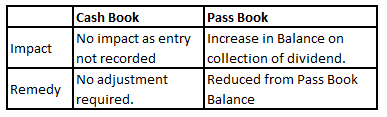Bank Reconciliation Statement Question 13 :

#### Favourable balance as per the pass book-interest allowed by the bank not recorded in cash book

If the Balance as per Pass Book is a (Cr.) of Rs. 11900 , interest of Rs. 725 ,allowed by the Bank would result an/a ____________ in Cr. balance ?

Explanation:

When interest is allowed by the Bank, the balance as per the Pass Book would have increased on allowance of such interest.

However, if such interest is not recorded in the cash book, the cash book balance would remain the same, although it should have increased.

To reconcile the two, such interest would be reduced from Pass Book balance to bring it at par with balance as per the Cash book.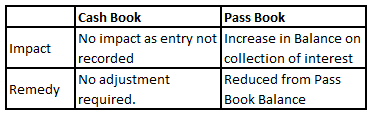#### Favourable balance as per the pass book-wrong credit granted by the bank

If the Balance as per Pass Book is a (Cr.) of Rs. 10000 , wrong credit of Rs. 4000 by the bank would result an/a ___________ in Cr. balance ?

Explanation:

When a Wrong credit is granted by the bank, the balance as per the Pass Book would have increased on such credit.

However, if such Credit is not recorded in the cash book, the cash book balance would not be impacted and remain the same.

To reconcile the two, such credit would be reduced from Pass Book balance to bring it at par with balance as per the Cash book.